# 机器学习算法 之逻辑回归以及python实现

1. 逻辑回归的相关原理说明
2. 通过python代码来实现一个梯度下降求解逻辑回归过程

# 逻辑回归(Logistic Regression)

m m $m$ 代表训练集中实例的数量

$n$$n$ 代表特征的数量

x(i) x ( i ) $x^{(i)}$ 表示第 i i $i$个训练实例，是特征矩阵的第i行，是一个向量

${x}_{j}^{\left(i\right)}$$x_j^{(i)}$ 表示特征矩阵中第 i i $i$行的第$j$$j$个特征，也就是第 i i $i$个训练实例的第$j$$j$个特征

y y $y$ 代表目标变量，也就是输出变量

$\left(x,y\right)$$(x,y)$ 代表训练集中的一个实例

(x(i),y(i)) ( x ( i ) , y ( i ) ) $(x^{(i)},y^{(i)})$ 代表第 i i $i$个观察实例

$h$$h$ 代表学习算法的函数，或者假设（hypothesis）

## 假设函数

z=θTX z = θ T X

g(z)=11+ez g ( z ) = 1 1 + e − z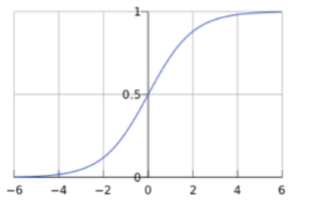hθ(x)=g(θTX)=11+eθTX h θ ( x ) = g ( θ T X ) = 1 1 + e − θ T X

P(y=1|x;θ)=hθ(x) P ( y = 1 | x ; θ ) = h θ ( x )
P(y=0|x;θ)=1hθ(x) P ( y = 0 | x ; θ ) = 1 − h θ ( x )

## 目标函数

P(y|x;θ)=(hθ(x))y(1hθ(x))1y P ( y | x ; θ ) = ( h θ ( x ) ) y ( 1 − h θ ( x ) ) 1 − y

L(θ)=i=1mP(y(i)|x(i);θ)=i=1m(hθ(x(i)))y(i)(1hθ(x(i)))1y(i) L ( θ ) = ∏ i = 1 m P ( y ( i ) | x ( i ) ; θ ) = ∏ i = 1 m ( h θ ( x ( i ) ) ) y ( i ) ( 1 − h θ ( x ( i ) ) ) 1 − y ( i )

l(θ)=logL(θ)=log(i=1m(hθ(x(i)))y(i)(1hθ(x(i)))1y(i))=i=1m[log(hθ(x(i)))+log(1hθ(x(i)))]=i=1m[y(i)log(hθ(x(i)))+(1y(i))log(1hθ(x(i)))] l ( θ ) = l o g L ( θ ) = l o g ( ∏ i = 1 m ( h θ ( x ( i ) ) ) y ( i ) ( 1 − h θ ( x ( i ) ) ) 1 − y ( i ) ) = ∑ i = 1 m [ l o g ( h θ ( x ( i ) ) ) + l o g ( 1 − h θ ( x ( i ) ) ) ] = ∑ i = 1 m [ y ( i ) l o g ( h θ ( x ( i ) ) ) + ( 1 − y ( i ) ) l o g ( 1 − h θ ( x ( i ) ) ) ]

J(θ)=1mi=1m[y(i)log(hθ(x(i)))+(1y(i))log(1hθ(x(i)))] J ( θ ) = − 1 m ∑ i = 1 m [ y ( i ) l o g ( h θ ( x ( i ) ) ) + ( 1 − y ( i ) ) l o g ( 1 − h θ ( x ( i ) ) ) ]

J(θ)θj=1mi=1m(hθ(x(i))y(i))x(i)j ∂ J ( θ ) ∂ θ j = 1 m ∑ i = 1 m ( h θ ( x ( i ) ) − y ( i ) ) x j ( i )

J(θ)θ=1mXT(Sigmoid(θX)y) ∂ J ( θ ) ∂ θ = 1 m X T ( S i g m o i d ( θ X ) − y )

## 决策边界

hθ(x)>=0.5 h θ ( x ) >= 0.5 $h_{\theta}(x) >=0.5$时，预测 y=1 y = 1 $y=1$,当 hθ(x)<0.5 h θ ( x ) < 0.5 $h_{\theta}(x) < 0.5$时，预测 y=0 y = 0 $y=0$

θTX>=0 θ T X >= 0 $\theta^TX >= 0$时，预测 y=1 y = 1 $y=1$,当 θTX<0 θ T X < 0 $\theta^TX < 0$时，预测 y=1 y = 1 $y=1$

θTX=0 θ T X = 0

# 梯度下降求解逻辑回归问题

import numpy as np
import pandas as pd
import matplotlib.pyplot as plt
import seaborn as sns
from sklearn.metrics import classification_report#这个包是评价报告

#exam1,exam2代表两次测试评分，也就是特征值x1,y1，admitted为录取结果，也就是y
data.head()  #打印前五行，看看
034.62366078.0246930
130.28671143.8949980
235.84740972.9021980
360.18259986.3085521
479.03273675.3443761

# 数据可视化
plt.show()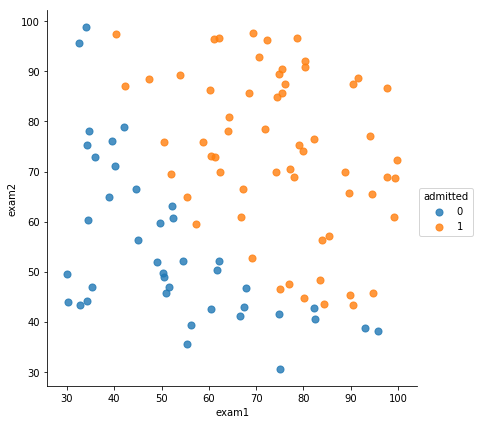### 数据初始化

#进行归一化处理，也就是特征缩放（注意，这块只是针对特征，也就是X）
def normalize_feature(df):
data_explame = df.iloc[:,:-1]
data_x = data_explame.apply(lambda column: (column - column.mean())/column.std())
data = pd.concat([data_x,df.iloc[:,-1]], axis=1)
return data

#插入x0列并转换为ndarray
def insert_x0_and_asmatrix(data):
ones = pd.DataFrame({'ones': np.ones(len(data))}) #生成一个m行1列的dataFrame
data = pd.concat([ones,data], axis=1)  #根据列，合并数据
return data.as_matrix()    #转化为ndarray进行返回

#刷新数据
def shuffleData(data):
np.random.shuffle(data)
return data

#读取特征
def get_X(data):
return data[:,:-1]

#读取输出变量
def get_Y(data):
return data[:,-1]


data = normalize_feature(data)
data.head()  #打印下特征缩放后的数据看看
0-1.5942160.6351410
1-1.817101-1.2014890
2-1.5313250.3594830
3-0.2806871.0809231
40.6880620.4909051
orig_data = insert_x0_and_asmatrix(data)  #加入x0列
orig_data[0:5]
array([[ 1.        , -1.59421626,  0.63514139,  0.        ],
[ 1.        , -1.81710142, -1.20148852,  0.        ],
[ 1.        , -1.53132516,  0.35948329,  0.        ],
[ 1.        , -0.28068724,  1.08092281,  1.        ],
[ 1.        ,  0.68806193,  0.49090485,  1.        ]])

shuffleData(orig_data)  #打乱样本次序
orig_data[0:5]
array([[ 1.        ,  0.96558389, -1.22094762,  1.        ],
[ 1.        ,  0.14514418,  1.04248696,  1.        ],
[ 1.        ,  0.74754551, -1.15162347,  1.        ],
[ 1.        , -0.5222529 , -1.64944586,  0.        ],
[ 1.        ,  0.08608101,  0.01976857,  1.        ]])

X = get_X(orig_data)
y = get_Y(orig_data)
theta = np.zeros(3)
X.shape,y.shape,theta
((100, 3), (100,), array([0., 0., 0.]))


### sigmoid函数

g(z)=11+ez g ( z ) = 1 1 + e − z

def sigmoid(z):
return 1/(1+np.exp(-z))

#看下sigmoid函数的函数图像
numbers = np.arange(-10,10,step=0.1)
plt.plot(numbers, sigmoid(numbers),'r')
plt.show()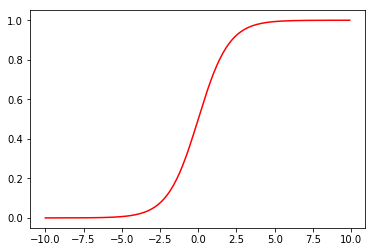### 计算代价函数

J(θ)=1mi=1m[y(i)log(hθ(x(i)))+(1y(i))log(1hθ(x(i)))] J ( θ ) = − 1 m ∑ i = 1 m [ y ( i ) l o g ( h θ ( x ( i ) ) ) + ( 1 − y ( i ) ) l o g ( 1 − h θ ( x ( i ) ) ) ]

#计算代价
def cost(theta, X, y):
return -np.mean(y * np.log(sigmoid(X @ theta))
+ (1-y) * np.log(1-sigmoid(X @ theta)))

cost(theta, X, y)
0.6931471805599453


### 计算梯度

J(θ)θj=1mi=1m(hθ(x(i))y(i))x(i)j ∂ J ( θ ) ∂ θ j = 1 m ∑ i = 1 m ( h θ ( x ( i ) ) − y ( i ) ) x j ( i )

J(θ)θ=1mXT(Sigmoid(Xθ)y) ∂ J ( θ ) ∂ θ = 1 m X T ( S i g m o i d ( X θ ) − y )

#计算梯度
return 1/len(X) * X.T @ (sigmoid(X @ theta) - y)

### 决策边界

θTX=0 θ T X = 0

def decision_fun(x,theta):
coef = -(theta/theta)
print(coef)
return coef + coef*x

def draw_decision_fun(theta):
x = np.arange(-2,2,step = 0.01)
y = decision_fun(x,theta)
plt.plot(x,y)
plt.xlim(-2,2)
plt.ylim(-2,2)
plt.title('Decision Boundary')
plt.show()

1. 直接设置迭代次数，次数到了停止迭代
2. 设置一个代价函数下降的阈值，如果本次和上一次迭代完后的代价函数的差值 如果小于这个阈值，则停止迭代
3. 设置梯度的阈值，如果某次迭代后，梯度小于阈值，则停止迭代

STOP_ITER = 0  #设置迭代次数
STOP_COST = 1  #设置目标函数下降的阈值

def stopCriterion(type, value, threshold):
#设定三种不同的停止策略
if type == STOP_ITER:        return value > threshold
elif type == STOP_COST:      return abs(value[-1]-value[-2]) < threshold
elif type == STOP_GRAD:      return np.linalg.norm(value) < threshold
#绘制代价函数图像
def drwcosts(costs):
fig, ax = plt.subplots(figsize=(12,4))
ax.plot(np.arange(len(costs)), costs, 'r')
ax.set_xlabel('Iterations')
ax.set_ylabel('Cost')

import time
def descent(data, theta, batchSize, stopType, thresh, alpha):
init_time = time.time()
i = 0; #迭代次数
k = 0; #batch
X = get_X(data)
y = get_Y(data)
costs = [cost(theta, X, y)]   #计算代价值

while True:
#根据不同的梯度下降算法（批量，随机，小批量），先计算梯度
k = k+batchSize
if k>= data.shape:
k = 0
shuffleData(data)   #重新打乱数据
X = get_X(data)
y = get_Y(data)
theta = theta - alpha * grad  #更新theta
costs.append(cost(theta, X, y)) #保存本次迭代后的目标值
i += 1  #迭代次数加1

#根据不同的停止策略，传入不同的value，来判断迭代是否结束
if stopType == STOP_ITER:
value = i
elif stopType == STOP_COST:
value = costs

if stopCriterion(stopType, value, thresh):  #如果满足停止策略，则停止迭代
break
print("theta:",theta)
print("迭代次数:",i-1)
print("最后求得的收敛的目标值:",costs[-1])
print("所用时间:",time.time() - init_time)
return theta, i-1, costs, grad, time.time() - init_time



### 不同的停止策略

theat, iter, costs, grad, durtime = descent(orig_data, theta, orig_data.shape, STOP_ITER, thresh=5000, alpha=0.0001)
drwcosts(costs)
theta: [0.04702107 0.13181832 0.11768083]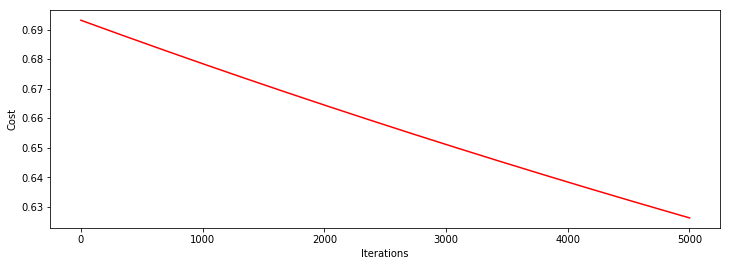heat, iter, costs, grad, durtime = descent(orig_data, theta, orig_data.shape, STOP_ITER, thresh=30000, alpha=0.0001)
drwcosts(costs)
theta: [0.21656628 0.60953923 0.54481303]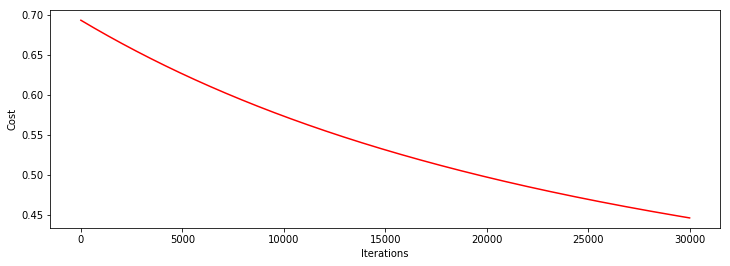heat, iter, costs, grad, durtime = descent(orig_data, theta, orig_data.shape, STOP_COST, thresh=0.000001, alpha=0.001)
drwcosts(costs)
theta: [0.88388452 2.24688547 2.04464801]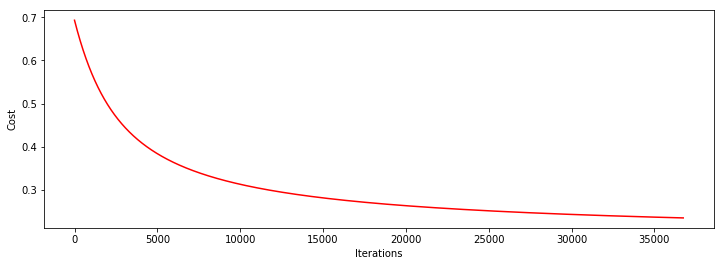heat, iter, costs, grad, durtime = descent(orig_data, theta, orig_data.shape, STOP_GRAD, thresh=0.01, alpha=0.001)
drwcosts(costs)
theta: [1.31220652 3.14902915 2.90906189]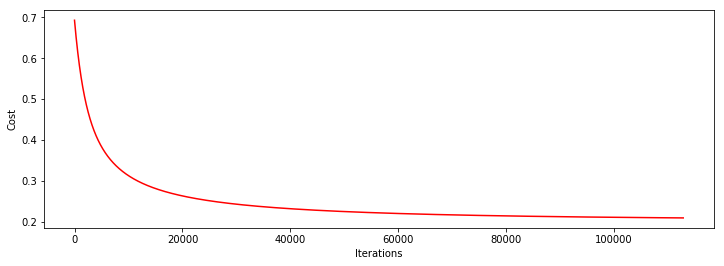### 对比不同的梯度下降方法

heat, iter, costs, grad, durtime = descent(orig_data, theta, 1,  STOP_GRAD, thresh=0.01, alpha=0.001)
drwcosts(costs)
theta: [0.67632327 1.78493605 1.61049596]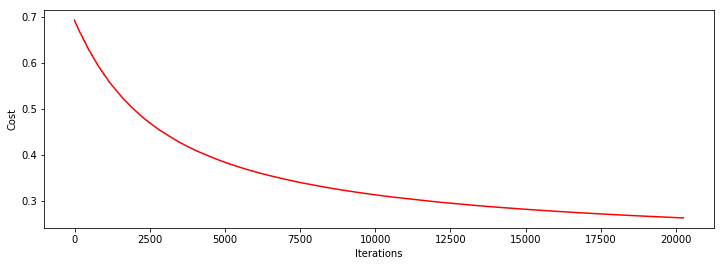heat, iter, costs, grad, durtime = descent(orig_data, theta, 64,  STOP_GRAD, thresh=0.01, alpha=0.001)
drwcosts(costs)
theta: [0.90249119 2.28523187 2.08373685]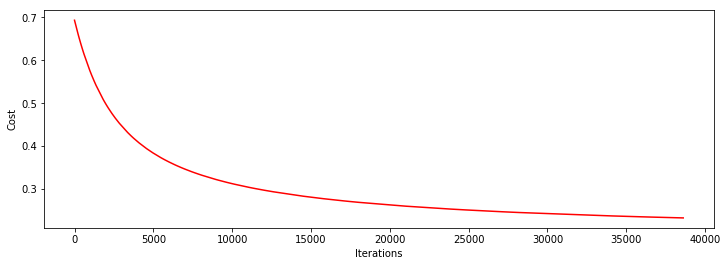### 用训练集预测和验证

def predict(X,theta):   #预测
prob = sigmoid(X @ theta)
return (prob >= 0.5).astype(int)
y_predict = predict(X, theat)  #得到训练集数据对应的预测结果
print(classification_report(y, y_predict))
#precision：精确度
#recall：召回率
#f1-score： f1值
             precision    recall  f1-score   support

0.0       0.88      0.88      0.88        40
1.0       0.92      0.92      0.92        60

avg / total       0.90      0.90      0.90       1009完美并不美

¥2 ¥4 ¥6 ¥10 ¥20余额支付 (余额：-- )扫码支付获取中扫码支付点击重新获取扫码支付1.余额是钱包充值的虚拟货币，按照1:1的比例进行支付金额的抵扣。
2.余额无法直接购买下载，可以购买VIP、C币套餐、付费专栏及课程。余额充值